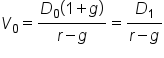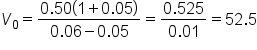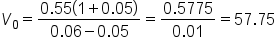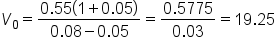+
Stock Valuation
Time Value of Money and Financial Securities
0
of 0 possible points

# Stock Valuation

Author: Sophia Tutorial
##### Description:

Calculate the value of a stock using the Constant Growth Model.

(more)### Developing Effective Teams

*No strings attached. This college course is 100% free and is worth 1 semester credit.

37 Sophia partners guarantee credit transfer.

299 Institutions have accepted or given pre-approval for credit transfer.

* The American Council on Education's College Credit Recommendation Service (ACE Credit®) has evaluated and recommended college credit for 32 of Sophia’s online courses. Many different colleges and universities consider ACE CREDIT recommendations in determining the applicability to their course and degree programs.

Tutorial

what's covered
In this lesson, you will learn about the value of stock and use the Constant Growth Model. Specifically, this lesson will cover:
1. Stock Valuation
2. Constant Growth Model
3. Relationship Between Dividend Payments and the Growth Rate

## 1. Stock Valuation

Just as we found the value of bonds by calculating the yield to maturity, the present value of future coupons, and maturity payments, the value of a stock is also found by examining the present value of expected future cash flows.

Stocks vary in attractiveness to different investors. This is called the clientele effect. At the end of an accounting period, a business can do one of two things with its net income:

• It can pay out dividends to the shareholders.
• It can reinvest it in the business.
Growth companies, often younger organizations, generally do not pay out dividends, but rather reinvest all of their profits into future growth. More mature companies will pay dividends because they are no longer growing at the same earlier rate.

big idea
In either case, we still can project the value of a stock based on our expectation of future dividends even if they are not being paid now.

## 2. Constant Growth Model

One familiar model that shows stock valuation is the Constant Growth Model. Here is the formula for the present value of a stock today that is a long series of the present value of expected future dividends:

###This calculation could be quite tedious depending on how far into the future we are expecting to receive the dividends. This calculation has been simplified into something called the constant growth model. The constant growth model allows us to value the price of a stock today on the basis of its next dividend divided by its required return minus its growth rate. Let's take a look at the simplified formula.

formula

Constant Growth ModelIn this formula:
• D0 is the last dividend that was paid
• r is the required return on a stock with the same level of risk as this company
• g is the expected growth rate that we expect to be constant in the future
hint
If we multiply D0 times 1 plus g, we have the next dividend, or D1; it is compounded by the growth rate.

### IN CONTEXT

Suppose we know the current annual dividend is \$0.50 a share, the required rate of return is 6%, and the growth rate is 5%. What current price should we expect?

 Current Annual Dividend 0.50 r (required return rate) 6.00% g (constant growth rate) 5.00%

We can use the formula, a web app, or a spreadsheet tool like Excel, to calculate this.Using this constant growth model, we get an expected price of \$52.50. So, if we are investing in the market, we may be looking at stocks with the same growth and required rate of return in dividends, as they might be undervalued and a better bargain for us.

### IN CONTEXT

What happens in the constant growth model for different situations? Suppose the board of directors votes to pay a \$0.55 annual dividend, rather than \$0.50. The required return rate and growth rate stay the same at 6% and 5%, respectively.

 Current Annual Dividend 0.55 r (required return rate) 6.00% g (constant growth rate) 5.00%

Let's use the constant growth model to calculate:Now the current price is \$57.75. Why is the price of the stock higher now? It is because the annual dividend is greater, so it is worth more to us.

### IN CONTEXT

Suppose the economy goes into a recession and the required return for the stock jumps to 8% because rates can increase in times of uncertainty. The currents annual dividend is still \$0.55 and the growth rate is 5%.

 Current Annual Dividend 0.55 r (required return rate) 8.00% g (constant growth rate) 5.00%

Again, we can use the constant growth model.Now the price falls; it plummets to \$19.25. Even though the dividend and constant growth rate did not change, the required return rate is much higher so the project value for that stock is only \$19.25.

## 3. Relationship Between Dividend Payments and the Growth Rate

Let's consider how this model works for companies that aren't paying dividends. Their price is still dependent upon the expectation of future dividends. Remember, a company can do one of two things with its net income:

• It can pay out dividends to the shareholders.
• It can reinvest it in the business.
Companies that are new or in a very high growth cycle will invest all of their net income back into the business. To keep the stock attractive when their future growth is not so rapid, they have to pay a dividend. Therefore, when a company runs through all of its growth opportunities, it will start paying dividends. The future value of those dividends is upon which the stock value is based.

What about companies that are not growing at a constant rate? Over the long-term life of a company, this is frequently the case. But we can use the model to estimate a value for a particular cycle.

EXAMPLE

If the company starts off with a period of high growth of 10%, we can use that growth rate for the early period. Later, if it flattens out for several years at 5%, use this rate as the growth rate for that period. After that, in maturity, if it only grows at 3%, use that as the rate, then combine the valuations of these three periods together.

summary
In this lesson, we learned the value of a share of stock is based on our expectations of future dividend payments in the present value of that cash flow. We can use the constant growth model to value the price of a stock today on the basis of its next dividend divided by its required return minus its growth rate. The relationship between dividend payments and the growth rate considers companies that are not paying dividends and companies that are not growing at a constant rate.

Best of luck in your learning!

Source: THIS CONTENT HAS BEEN ADAPTED FROM LUMEN LEARNING'S “Stock Valuation” TUTORIAL.

Formulas to Know
Constant Growth ModelRating